## Example Questions

### Example Question #1 : How To Find The Length Of The Side Of A Quadrilateral

Find the length of a rectangle if the area is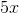, and the width is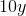.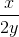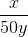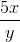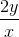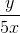Explanation:

Write the formula to solve the area of a rectangle.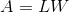Substitute the dimension and area to solve for the length.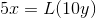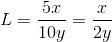### Example Question #2 : How To Find The Length Of The Side Of A Quadrilateral

If the perimeter of a square is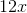and the area is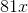, what is the side length of the square?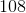Explanation:

The square has 4 equal sides.  Let's assume a side is.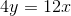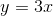Each side of the square has a length of.

The area of the square is: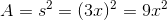Substituteinto the area to solve for.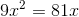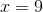Since one side of the square is, substitute the value ofto determine the length of the square side.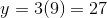### Example Question #3 : How To Find The Length Of The Side Of A Quadrilateral

Find the side of a square if the area is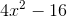.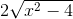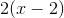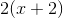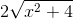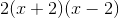Explanation:

The area of a square is: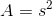Substitute the area and solve for the side.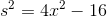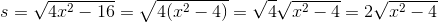The quantity inside the square root may not be factorized in attempt to eliminate the square root!

The side length of the square is:### Example Question #4 : How To Find The Length Of The Side Of A Quadrilateral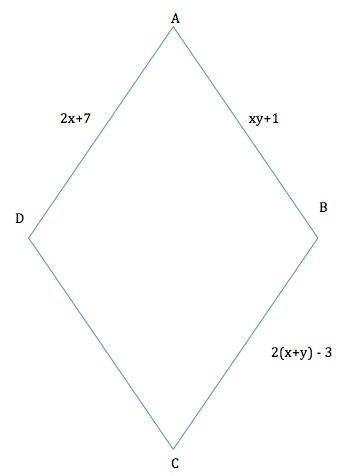Find the perimeter of the rhombus above.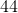Explanation:

By definition, a rhombus is a quadrilateral with four equal sides whose angles do not all equal 90 degrees. To find the perimeter, we must find the values of x and y. In order to do so, we must set up a system of equations where we set two sides equal to each other. Any two sides can be used to create these systems.

Here is one example:

Eq. 1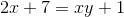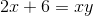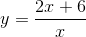Eq. 2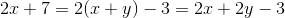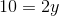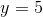Now we pluginto the first equation to find the value of: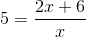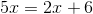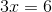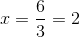Plugging these values into any of the three equations will give us the length of one side equaling 11.

Since there are four sides,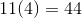.